# C Program to Convert Decimal to Hexadecimal

In this tutorial, we will learn how to create a program in C that converts any given decimal number (provided by the user at run-time) into its equivalent hexadecimal value. We have also created a user-defined function that does the same job of converting a decimal number to a hexadecimal number.

Before going through the program, if you are not aware of

• Decimal Number
• The Decimal to Hexadecimal Conversion Process

Then refer to "Decimal to Hexadecimal Conversion: A Step-by Process." Let's move on to the program.

## Decimal to hexadecimal in C

To convert a decimal number to a hexadecimal number in C programming, you have to ask the user to enter the decimal number as input and then convert it into its equivalent hexadecimal value, as shown in the program given below. The question is, "Write a program in C that converts decimal to hexadecimal." Here is its answer.

```#include<stdio.h>
#include<conio.h>
int main()
{
int decnum, rem, i=0;
char hexnum;
printf("Enter any decimal number: ");
scanf("%d", &decnum);
while(decnum!=0)
{
rem = decnum%16;
if(rem<10)
rem = rem+48;
else
rem = rem+55;
hexnum[i] = rem;
i++;
decnum = decnum/16;
}
printf("\nEquivalent Value in Hexadecimal = ");
for(i=i-1; i>=0; i--)
printf("%c", hexnum[i]);
getch();
return 0;
}```

Because the above program was built and run using the Code::Blocks IDE, you will receive the following output after a successful build and run. This is the first snapshot of the sample run: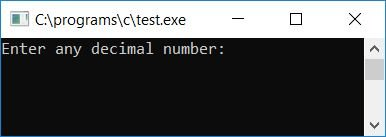Now supply any decimal number as input, say 172, and press the ENTER key to see the equivalent hexadecimal value as output. Here is the second snapshot of the sample run: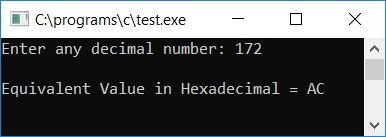Here is another sample run. This is the final snapshot of the sample run: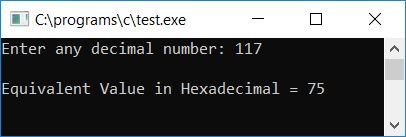#### Program Explained

• At program runtime, receive any decimal number entered by the user.
• Create a while loop with the condition decnum!=0. Here, the decnum variable holds the decimal number value entered by the user at run-time.
• Let's suppose that the user has entered 172 as input.
• Therefore, at the first run of the while loop, the condition decnum!=0 or 172!=0 evaluates to true. Therefore, program flow goes inside the loop.
• And decnum%16 or 172%16 (we will get a remainder of 12 because while dividing the number 172 (dividend) by 16 (divisor), we will get 10 as the quotient and 12 as the remainder) or 12 gets initialized to the rem variable.
• Now, using the if statement, determine whether the value inside the rem variable is less than 10, because if it is less than 10, the value can range from 0, 1, 2, ..., 8, 9. And we all know that the ASCII codes for 0 through 0 are 48, 49, 50, ..., 56, 57. Therefore, we have to apply the addition with 48 to the value available in the rem variable.
• Because if rem is 0, then rem+48 or 0+48 or 48 gets initialized to rem (which is the ASCII code for 0). Or if rem holds the value 4, then rem+48 or 4+48 or 52 gets initialized to rem, which equals the ASCII code for 4.
• And finally, rem (the ASCII code value) gets initialized to hexnum[i] (i holds 0 as its initial value) or hexnum.
• If the value of rem is greater than 10, the value in the rem variable can range between 10, 11, 12, ..., 14, 15.
• In the hexadecimal number system, 10 is represented by the letter A, 11 is represented by the letter B, ..., 15 by the letter F. Furthermore, the ASCII codes for A, B, C,..., E, F are 65, 66, 67,..., 69, 70.
• Therefore, rem+55 gets initialized to rem. We have added the value of rem with 55, as if the value of rem is 10, then we have to initialize the ASCII code of A, as 10 is represented by A. And the ASCII code of A is 65. As a result, the ASCII code of 0, 1, 2, ..., E, or F is initialized to hexnum[i] or hexnum. Therefore, at the first run of the while loop. We got a remainder value of 12, therefore rem+55 or 12+55 or 67 gets initialized to rem, and then rem gets initialized to hexnum[i] or hexnum.
• Then the value of i gets incremented and becomes 1. And decnum/16 or 172/16 or 10 gets initialized to decnum.
• Now at the second run of the while loop, the condition decnum!=0 or 10!=0 evaluates to true, therefore, program flow goes inside the loop again and does the similar job as described in the above steps.
• We successfully stored the equivalent hexadecimal value of the given decimal number one by one after exiting the while loop.
• Therefore, to print the number in hexadecimal form, we have to create a for loop that runs from one less than the current value of i to 0.
• Because we increased the value of i in the previous run. Because the last run indicates that decnum contains 0, the condition (decnum!=0 or 0!=0) evaluates to false, so we must subtract the current value of i from 1.
• Print all the digit of the hexnum[] array one by one, from the last digit to the first digit.
• At program run-time, we have successfully printed the equivalent hexadecimal value of the given decimal value.

### Decimal to Hexadecimal in C without the Modulus Operator

Now let's create the same program but without using any modulus operators. That is, we have to find out the remainder without using the modulus operator. Therefore, to find the remainder, we have divided the number present in decnum by 16 and stored its quotient value inside a variable, say temp, then again multiplied the quotient value with 16 and stored the multiplication result in a variable, say chck. Subtract chck from decnum (decnum - chck) and assign the result to the rem variable, which will store the current remainder.

For example, if the user enters 172 as input, decnum/16 or 10 is initialized to temp on the first run. Then, to check, temp*16, 10*16, or 160 is initialized to chck. Now decnum-chck, 172-160, or 12 is initialized to rem, which is this time the remainder. In this way, we have replaced the modulus operator with three lines of code, as shown in the program given below:

```#include<stdio.h>
#include<conio.h>
int main()
{
int decnum, hexnum, temp, chck, i=0, rem;
printf("Enter any Decimal number: ");
scanf("%d", &decnum);
while(decnum!=0)
{
temp = decnum/16;
chck = temp*16;
rem = decnum - chck;
if(rem<10)
rem = rem+48;
else
rem = rem+55;
hexnum[i] = rem;
i++;
decnum = temp;
}
for(i=i-1; i>=0; i--)
printf("%c", hexnum[i]);
getch();
return 0;
}```

Here is the final snapshot of the sample run: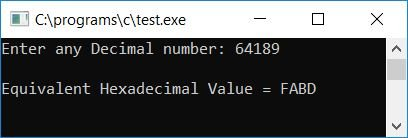### Decimal to Hexadecimal in C using a User-Defined Function

Now let's create a user-defined function named DecToHex() that takes one argument (the decimal number entered by the user). Here we have declared the variable i  and the array hex[] outside both the functions main() and DecToHex() to make them known for both functions. Here, the variable i is declared as a static variable because a static variable remembers its previous value. One last thing is that we have not initialized the static variable i with 0 (which is required), because by default, a static variable holds 0 as its initial value after being declared. The rest of the thing is similar and easy to understand.

```#include<stdio.h>
#include<conio.h>
void DecToHex(int dec);
static int i;
char hex;
int main()
{
int decnum;
printf("Enter any decimal number: ");
scanf("%d", &decnum);
DecToHex(decnum);
printf("\nEquivalent Value in Hexadecimal = ");
for(i=i-1; i>=0; i--)
printf("%c", hex[i]);
getch();
return 0;
}
void DecToHex(int dec)
{
int rem;
while(dec!=0)
{
rem = dec%16;
if(rem<10)
rem = rem+48;
else
rem = rem+55;
hex[i] = rem;
i++;
dec = dec/16;
}
}```

Below is the final snapshot of the sample run of the above program: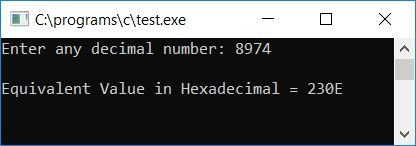#### The same program in different languages

« Previous Program Next Program »